# Nemirovski’s acceleration

I will describe here the very first (to my knowledge) acceleration algorithm for smooth convex optimization, which is due to Arkadi Nemirovski (dating back to the end of the 70’s). The algorithm relies on a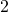-dimensional plane-search subroutine (which, in theory, can be implemented in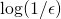calls to a first-order oracle). He later improved it to only require a-dimensional line-search in 1981, but of course the breakthrough that everyone knows about came a year after with the famous 1982 paper by Nesterov that gets rid of this extraneous logarithmic term altogether (and in addition is based on the deep insight of modifying Polyak’s momentum).

Letbe a-smooth function. Denote. Fix a sequence, to be optimized later. We consider the “conjugate” point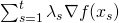. The algorithm simply returns the optimal combination of the conjugate point and the gradient descent point, that is: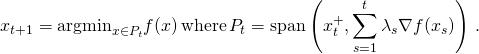Let us denote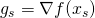and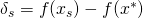for shorthand. The key point is that, and in particular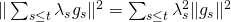. Now recognize that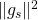is a lower bound on the improvement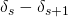(here we use thatis better than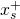). Thus we get: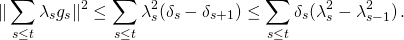In other words if the sequence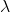is chosen such that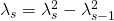then we getThis is good because roughly the reverse inequality also holds true by convexity (and the fact thatso):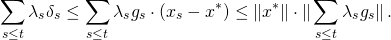So finally we get, and it just remains to realize that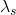is of orderso that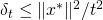.

This entry was posted in Optimization. Bookmark the permalink.

## 8 Responses to "Nemirovski’s acceleration"

•••••••